# Solving Linear Equations - Algebra Math Review

math tutorials > linear equations math tutorial

## An algebraic equation contains at least one variable (e.g. x, y or z) and an equal sign.

In a linear equation the variable has a power of 1.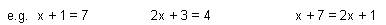To solve a linear equation you need to find the value of the variable which makes the equation true.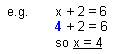## Solving difficult linear equations.

1. When an operation is performed on one side of the equation, the same operation must be performed on the other side of the equation.
2. Start with the numbers furthest from the variable, and work towards the variable.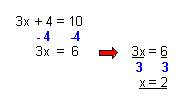3. The equation may need to be rearranged to have all the variables on one side of the equal sign and all the numbers on the other.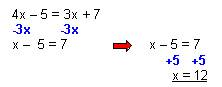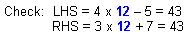## Algebra problems for you to try.

1.  –3x + 5 = 41

2.  7x – 8 = 20

3.  4x – 5 = x + 10

Solutions: 1.  x = -12        2.  x = 4         3.  x = 5

## Things to remember.

Rules for multiplying and dividing integers apply.  When multiplying or dividing a pair of numbers, like signs (+ and +, or – and - ) give a positive answer and unlike signs (+ and -) give a negative answer.